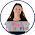# Trig Ratios INB Pages

At the recommendation of a colleague, I taught trig ratios a little bit differently this year.  I ended up spending a week on trig ratios.  The first day, I only introduced the ratios.  The second day, I actually had students set them up into an equation.  The third day, we did inverse trig ratios.  The last two days were spent on application and word problems.

It. Was. Awesome.

I’m so glad I tried it.  In the past, I’ve always done some kind of investigation about the tangent ratio, then did the other two ratios the next day.  It was okay, but the investigation didn’t really do much for my students and it all seemed like a waste of time.  Changing it up was just what I needed.

The first day, all I did was talk about the ratios.  We completed this page explaining what each ratio was and labeled a right triangle.

Then, I had students glue in this triangle that we labeled.  I had them find the missing side and write the ratios for each angle.  I showed them the relationship between tanA & tanB, cosA & sinB, and sinA & cosB.  Then, we made a “magic book” were students had to write six ratios (three for each angle) for each triangle.

The next day, we actually used the ratios to solve problems.  I used my trig ratios foldable.  I also helped them trouble shoot potential problems on the calculator.

On the third day, we solved for angles using the inverse trig ratios.  I used by inverse trig ratios foldable.  By this time, most of my kids were pros.

On the fourth day, I used these interactive notebook pages by Secondary Math Shop.  They explained the angles of elevation and depression and then had two practice problems each.
PSA: Whenever you teach this, always remind students where a shadow is.  So many of them want to make the shadow the hypotenuse.  I try to help them fix the problem themselves by asking, “If you want to see your shadow, where do you look?”

The next day, we reviewed angles of depression and elevation using a stations maze.  My students did SO WELL on this.  Several of them have said it was their favorite thing so far this year!1.1.